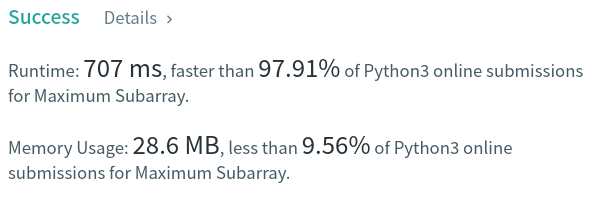Algorithm Puzzles everyday every week sometimes: Maximum Subarray

## Puzzle

Puzzle from leetcode:

Given an integer array nums, find the contiguous subarray (containing at least one number) which has the largest sum and return its sum.

A subarray is a contiguous part of an array.

## Solution

TC: O(n)